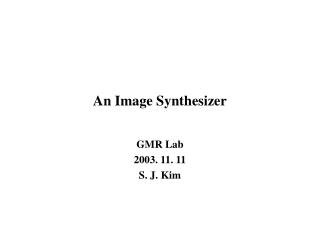DownloadDownload PresentationAn Image Synthesizer

# An Image Synthesizer

Télécharger la présentation## An Image Synthesizer

- - - - - - - - - - - - - - - - - - - - - - - - - - - E N D - - - - - - - - - - - - - - - - - - - - - - - - - - -
##### Presentation Transcript

1. An Image Synthesizer GMR Lab 2003. 11. 11 S. J. Kim

2. Introduction • A motive • Out of some experiments into developing efficient naturalistic looking textures. A Pixel Stream Editing Language (PSE) • Use PSE • Some rather convincing naturalistic detail with surprisingly simple • Efficient algorithms.

3. A Pixel Stream Editing Language • A Pixel Stream Editor (PSE) • Simply a filter Input image Output image Convert At every pixel • Supported all of the familiar programming constructs • All operators will work on scalars or vectors

4. Interactive design cycle • Edit PSE program • Run it on a low resolution image • View the results on a color monitor

5. Space Functions and Solid Texture • The domain is entirety of (x, y, z) space : “space function” • Any space function Solid material • Solid Texture – the object out of the material (“ sculpted”) • This approach has several advantages over texture mapping: • Shape and texture become independent. The texture does not need to be “fit” onto the surface. If we change the shape or carve a piece out of it, the appearance of the solid material will accurately change. • As with all procedural textures, the database is extremely small.

6. Noise ( ) • A scalar valued function (takes a three dimensional vector) • Properties: • Statistical invariance under rotation • A narrow bandpass limit in frequency • Statistical invariance under translation • A good texture modeling primitive –> create surfaces (with desired stochastic characteristics at different visual scales)

7. Integer lattice • Consider the set of all points in space whose x, y, and z coordinates are all integer valued • If [x, y, z] is on the integer lattice, we define Noise([x, y, z]) = d[x, y, z].

8. By evaluating Noise() at visible surface points of simulated objects – create a simple “random” surface texture color = white * Noise(point) To map different ranges of values into different colors color = Colorful( Noise(k *point))

9. Dnoise( ) • The vector valued differential of the Noise() signal • Defined by the instantaneous rate of change of Noise() along the x, y, and z directions, respectively A simple way of specifying normal perturbation normal += Dnoise(point)

10. By using functions of Noise() to control the amount of Dnoise() perturbation -> simulate various types of surface Use these in turn to design other types of surface

11. f = 1 while f < pixel_freq nomal += Dnoise(f * point) f *= 2

12. Marble – An Example of a Solid Texture • Use Noise() to create function turbulence() • Use turbulence() to simulate the appearance of marble function boring_marble(point) x = point return marble_color(sin(x)) function marble(point) x = point + turbulence(point) return marble_color(sin(x))

13. Fire • Using turbulence( ) • Ex) a solar corona • norm() scalar length of a vector • direction() the (unit length) direction of a vector • frame global time variable • Modeled as a smooth flow smooth_corona(point-center) function smooth_corona(v) radius = norm(v) return color_of_emission(radius) function corona(v) radius = norm(v) dr = turbulence(v) return color_of_corona(radius + dr) Function moving_corona(v) radius = norm(v) dr = turbulence(v – frame * direction(v)) return color_of_corona(radius + dr)

14. Water • Create the appearance of waves on a surface • Linear wave front • Deficiency – a self-replicating pattern • Use spherical wave fronts eminating from point source centers normal += wave(point-center) function wave(v) return direction(v) * cycloid(norm(v)) function makewaces(n) for i in [1 .. n] center[i] = direction(Dnoise(i*[100 0 0])) return center if begin_frame center = makewaves(20) for c in center normal += wave(point – c)

15. Assign a random frequency f to each center -> the last of the procedure then becomes: normal += wave((point - c) * f) / f function moving_wave(v, Dphase) return direction(v) * cycloid(norm(v) – frmae * Dphase)

16. Other Examples – Clouds and Bubbles • Using the PSE • Clouds • Created by composing a color spline function with turbulence() • The reflection and refraction from the bubble • Using simple vector valued functions to modify an incoming direction vector • The appearance of variable bubble thickness • Simulated by multiplying turbulence() Color *= 1 + sin([rfreq gfreq bfreq] * turbulence(point))